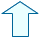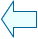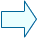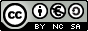The mental calculation of the square root of a number Square roots

The mental calculation of the square root of a number.

The square root is the inverse of the square. We can obtain the square root of a number by trying to find which number turns out to be the radicand when it is multiplied by itself.
This method is used with simple numbers, like natural numbers smaller than 100.

Esta unidad interactiva requiere la máquina virtual de Java J2RE.

Calculate the whole square root of the number shown, type it in the control box below and hit Enter.

When you get it right, the word CORRECT will appear in the window. But if you type a wrong number, the word will not appear.

Every time you click on Init a new perfect natural square number will appear in the radicand, the square root of which is another natural number.
Try to find the square root of several numbers.

Esta unidad interactiva requiere la máquina virtual de Java J2RE.

The radicand is a  natural number that is probably not a perfect square, so its square root has a remainder.

Find the whole square root by default, that is, you have to find a natural number whose square is closest to the radicand, but does not exceed it.

Esta unidad interactiva requiere la máquina virtual de Java J2RE.

The radicand is a natural number or a perfect square decimal number, that has got only one digit different from 0. Finding its square root is easy.

 To type a decimal number in the control box below you have to use the dot instead of the comma.

The square root of a positive number has two real solutions and both solutions -the positive and the negative- are correct.
The square root of a negative number does not have a real solution because there is no positive or negative number that -when it is multiplied by itself- has a negative result.

 Esta unidad interactiva requiere la máquina virtual de Java J2RE. Take the numbers below to the corresponding circle. In this window the radicands of the six square roots are perfect square numbers. The numbers below are the solutions.Eduardo Barbero CorralSpanish Ministry of Education. Year 2007